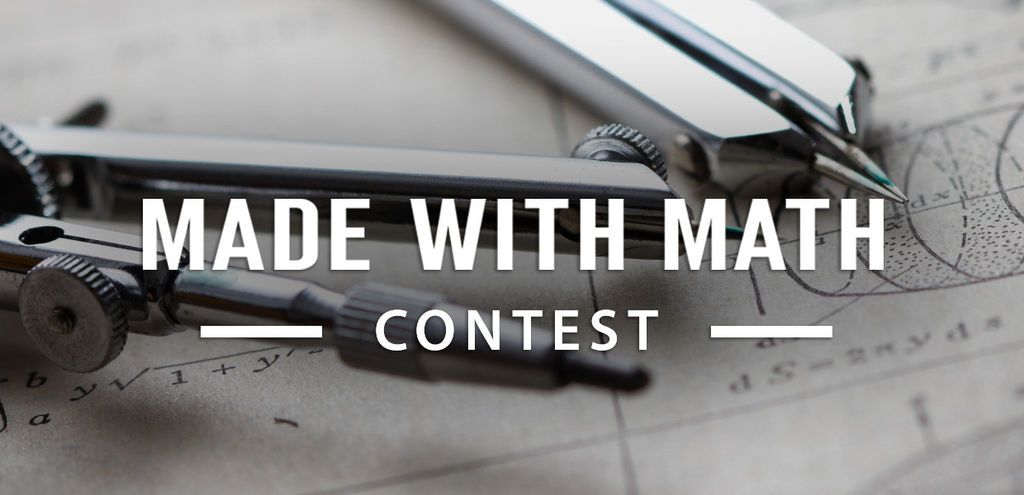# Guess My Number

1,017

9

8

This is a simple number guessing game.

Ask your friend to think of a number (1-15) - don't tell

Show them 4 lists of numbers asking them to say yes or no if the number is on the list.

Tell them the number they were thinking of.

Easy.

• Pencil
• Paper
• Scissors
• A friend

### Teacher Notes

Teachers! Did you use this instructable in your classroom?
Add a Teacher Note to share how you incorporated it into your lesson.

## Step 1: The Lists of Numbers and Guesing

Building the game:

1. Cut a piece of paper into 4 strips. (Leave enough room to write 8 numbers).
2. Write the following lists, one on each strip of paper
 List A: 1, 3, 5, 7, 9, 11, 13, 15 List B: 2, 3, 6, 7, 10, 11, 14, 15 List C: 4, 5, 6, 7,12, 13, 14, 15 List D: 8, 9, 10, 11, 12, 13, 14, 15

Playing the game:

• Tell your fried to guess a number: (1-15) (don't tell).
• Show them each strip one and a time and ask if the number is on that strip.
• Every time they say "Yes" add the first number in the list to a total.
• After all lists have been reviewed, tell them the total of the first numbers of all the lists their number appeared in.

Example Game:

My number is 12.

I say "No" to List A

I say "No" to List B

I say "Yes" to List C (4 is first number)

I say "Yes" to List D (8 is first number)

4+8 =12 so my friend tells me 12 is my number.

## Step 2: Why This Works

Although its not requires to understand this to use the lists:

Its simply Binary or Base2. As you may be aware binary is a list of 1s and 0s.

If we look at our numbers 1-15 and the binary number for the same value.

```Decimal : Binary
lists DCBA
1 = 0001
2 = 0010
3 = 0011
4 = 0100
5 = 0101
6 = 0110
7 = 0111
8 = 1000
9 = 1001
10 = 1010
11 = 1011
12 = 1100
13 = 1101
14 = 1110
15 = 1111
```

In binary or base 2 the rightmost number is 1, the next is 2 then 4 followed by 8. Think of the strips of paper as columns to hold numbers down the list. Every row with a 1 in the column would go on that strip. The first list "List A" would hold 1,3,5,7,9,11,13,15 because the right most number is a one. The number 7(111) would be in List A, List B and List C because there are 1's in the first, second and third places. In binary 111 would be in decimal 4+2+1 = 7.

Challenge yourself:

Want to take the numbers higher than 15? Binary number make a pattern, if we added a List E we could go to 31. The first number in List E would be 16. If we added a List F we could go to 63 and the first number in that list would be 32.

Here is a list for 1-31. Try it!!

```List A	List B	List C 	List D	List E
1	2	4	8	16
3	3	5	9	17
5	6	6	10	18
7	7	7	11	19
9	10	12	12	20
11	11	13	13	21
13	14	14	14	22
15	15	15	15	23
17	18	20	24	24
19	19	21	25	25
21	22	22	26	26
23	23	23	27	27
25	26	28	28	28
27	27	29	29	29
29	30	30	30	30
31	31	31	31	31
```

There are 10 types of people, those who understand binary and those that do not!

### If you like this Instructable please Vote for it!!This is an entry in the